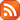Subscribe by RSS

# Department Colloquium

Fri., Jul. 14, 2023 3:30 p.m.

Location: RIC 208

Speaker: Projesh Nath Choudhury, IIT Gandhinagar

Title:  Blowup-polynomials of graphs (102 kB)Abstract:

Given a finite simple connected graph G = (V,E), we introduce a novel invariant which we call its blowup-polynomial pG(nv : vV). To do so, we compute the determinant of the distance matrix of the graph blowup, obtained by taking nv copies of the vertex v, and remove an exponential factor.
• First: we show that as a function of the sizes nv, pG is a polynomial, is multi-affine, and is real-stable.
• Second: we show that the multivariate polynomial pG is intimately related to the characteristic polynomial qG of the distance matrix DG, and that it fully recovers G whereas qG does not.
• Third: we obtain a novel characterization of the complete multi-partite graphs, as precisely those whose "homogenized" blowup-polynomials are Lorentzian/strongly Rayleigh.
• Finally: we show how to obtain from pG a novel delta-matroid for every graph. We also provide a second delta-matroid for every tree, which too is hitherto unexplored, but whose construction does not extend to all graphs.

(Joint with Apoorva Khare.)